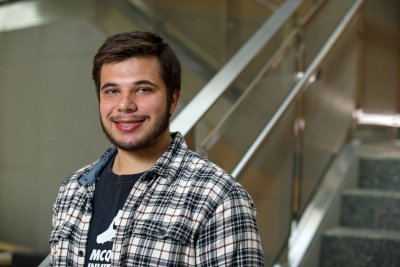Approximate Sampling Distribution of Sample Correlation Coefficient Under Type I CensoringStudent: Jacob Tarnowski (St. John Fisher College)
Research Mentor: Scott Linder (OWU Department of Mathematics and Computer Science

Suppose a random sample of size $n$ is selected from a bivariate normal population and exposed to Type I (time constrained) censoring on one of the variates, so that cases associated with the values of one of the variates beyond time T are censored.  The presence of censoring in this context renders intractable the sampling distribution of the sample correlation coefficient.  Using simulation, we systematically examine the impact of censoring on the sampling distribution of the absolute correlation coefficient, $|r|$. We propose approximation of the sampling distribution of this statistic by the Beta distribution, whose parameters are determined as functions of the experimental conditions ($n$, proportion censored ($\theta$), and $\rho$).  These functions are regression models fit to the average maximum likelihood parameter estimates obtained through simulation.  We examine the goodness-of-fit of this approximate sampling distribution, and also consider the relative error of estimation of percentiles of this distribution commonly necessary for inference.

In many industrial or clinical settings, subjects in experiments are time censored - that is, the experiment ends at a particular time and any events that have not occurred by that time are not observed.  In these settings, the traditional inferential statistical methods applied to complete samples containing independent observations are not appropriate because censoring impacts the sampling distributions of associated statistics.  In this work we examine the impact of time censoring (Type 1 censoring) on the sampling distribution of the sample correlation coefficient, and we propose a simple method for approximating it.  This approximation allows for more appropriate inference about the correlation between two variables in such a setting.